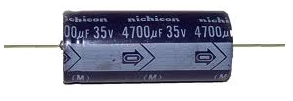﻿ What is the Nominal Value of a Component

# What is Nominal Value of a Component?

The nominal value of a component is the value that a component is rated for, or its list value.

This is the value that the component will have either directly on it or through some color-coded system.

For example, the nominal value of the capacitor below is 4700µF.However, just because a component has a nominal value doesn't mean it will be that value actually. The actual value may be different and how much it varies depends on the tolerance of the component and whether it's in good condition.

If a component varies by a tolerance of 20%, its nominal value can vary by being 20%; it can be 20% higher or lower than its nominal value. So if a capacitor has a tolerance of 4700µF and has a tolerance of 20%, the actual value in a circuit can be between 3760µF and 5640µF. So this is the difference between a component's nominal value and its actual value.